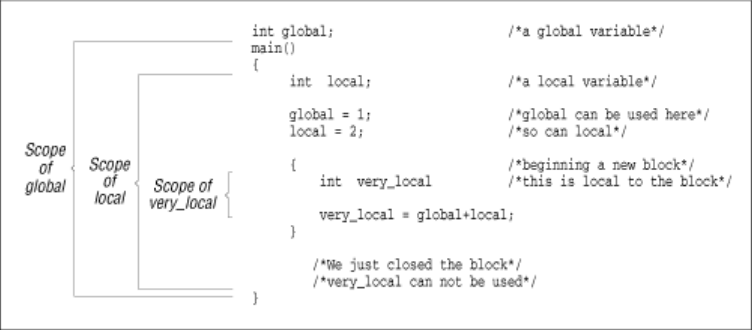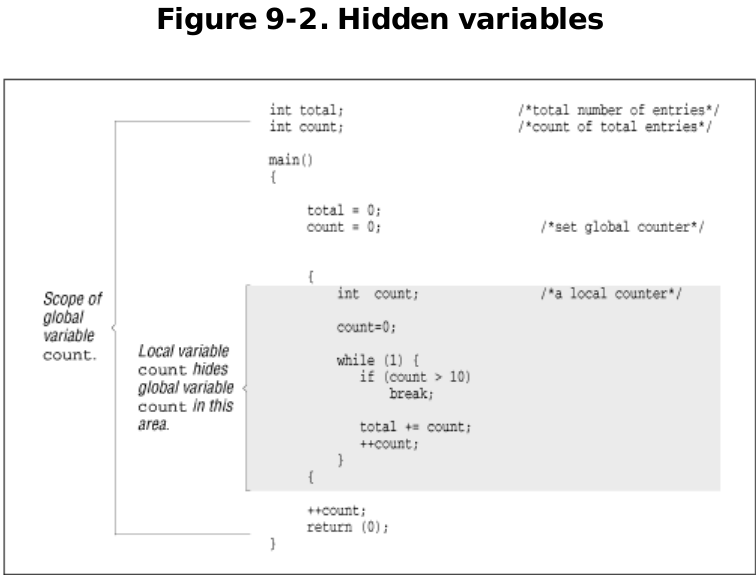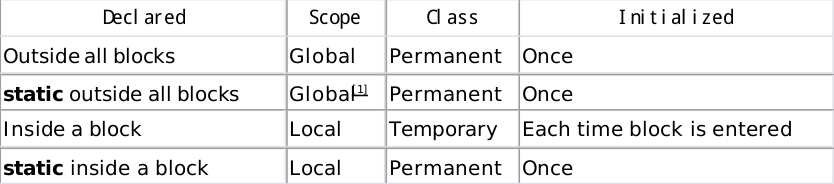# Chapter 9 Notes – Practical C Programming, 3rd Edition By Steve Oualline

Chapter 9 introduces local variables, functions, and parameters.

A big vim tip here for prog editing/writing:

Lesson 4.3: MATCHING PARENTHESES SEARCH
** Type % to find a matching ),], or } . **

1. Place the cursor on any (, [, or { in the line below marked —>.

2. Now type the % character.

3. The cursor will move to the matching parenthesis or bracket.

4. Type % to move the cursor to the other matching bracket.

5. Move the cursor to another (,),[,],{ or } and see what % does.

—> This ( is a test line with ('s, ['s ] and {'s } in it. ))
NOTE: This is very useful in debugging a program with unmatched parentheses!

2. Press G to move you to the bottom of the file.
Type gg to move you to the start of the file.

All variables have two attributes: scope and class. The scope of a variable is the area of the program in which the variable is valid. A global variable is valid everywhere (hence the name global), so its scope is the whole program. A local variable has a scope that is limited to the block in which it is declared and cannot be accessed outside that block.You can declare a local variable with the same name as a global variable. Normally, the scope of the variable count (first declaration) would be the whole program. The declaration of a second local count takes precedence over the global declaration inside the small block in which the local count is declared.The variable count is declared as both a local variable and a global variable.

Normally, the scope of count (global) is the entire program; however, when a variable is declared inside a block, that instance of the variable becomes the active one for the length of the block. The global count has been hidden by the local count for the scope of this block. The shaded block in the figure shows where the scope of count (global) is hidden.

A problem exists in that when you have the statement:

count = 1;

you cannot tell easily to which count you are referring. Is it the global count, the one declared at the top of main, or the one in the middle of the while loop? You should give these variables different names, like total_count , current_count , and item_count.

The class of a variable may be either permanent or temporary. Global variables are always permanent. They are created and initialized before the program starts and remain until it terminates. Temporary variables are allocated from a section of memory called the stack at the beginning of the block. If you try to allocate too many temporary variables, you will get a "Stack overflow" error. The space used by the temporary variables is returned to the stack at the end of the block. Each time the block is entered, the temporary variables are initialized.

The size of the stack depends on the system and compiler you are using. On many UNIX systems, the program is automatically allocated the largest possible stack.

Local variables are temporary unless they are declared static.

/*vars.c*/

#include <stdio.h>

int main()

{

int counter;

/* loop counter */

for (counter = 0; counter < 3; ++counter)

{

int temporary = 1; /* A temporary variable */

static int permanent = 1; /* A permanent variable */

printf("Temporary %d Permanent %d\n",

temporary, permanent);

++temporary;

++permanent;

}

return (0);

}

\$ ./vars

Temporary 1 Permanent 1

Temporary 1 Permanent 2

Temporary 1 Permanent 3Functions allow us to group commonly used code into a compact unit that can be

used repeatedly. We have already encountered one function, main. It is a special

function called at the beginning of the program. All other functions are directly or

indirectly called from main.

Suppose we want to write a program to compute the area of three triangles.

We could write out the formula three times, or we could create a function to do the work.

(Content summary:

printf("Triangle #1 %f\n", triangle(1.3, 8.3));

printf("Triangle #2 %f\n", triangle(4.8, 9.8));

printf("Triangle #3 %f\n", triangle(1.2, 2.0));

return (0);

)

Each function should begin with a comment block containing the following:

Name Name of the function

Description Description of what the function does

Parameters Description of each of the parameters to the function

Returns Description of the return value of the function

Our function to compute the area of a triangle begins with:

/*********************************************

* triangle — Computes area of a triangle.

*

* Parameters

*

* width — Width of the triangle. *

* height — Height of the triangle. *

*

* Returns

*

area of the triangle.

*

*********************************************/

/*tri-sub.c*/

#include <stdio.h>

/********************************************

* triangle — Computes area of a triangle. *

*

*

* Parameters

*

* width — Width of the triangle. *

* height — Height of the triangle. *

*

*

* Returns

*

*

area of the triangle.

*

********************************************/

float triangle(float width, float height)

{

float area;

/* Area of the triangle */

area = width * height / 2.0;

return (area);

}

int main()

{

printf("Triangle #1 %f\n", triangle(1.3, 8.3));

printf("Triangle #2 %f\n", triangle(4.8, 9.8));

printf("Triangle #3 %f\n", triangle(1.2, 2.0));

return (0);

}

Declaring a prototype for a function without parameters is a little tricky. You can't

use the statement:

int next_index();

because the C compiler will see the empty parentheses and assume that this is a

Kernighan & Richie-style function declaration. The keyword void is used to indicate an empty parameter list. So the prototype for our next_index function is:

int next_index(void);

void is also used to indicate that a function does not return a value.

Question 9-1 : Example 9 -4 should compute the length of a string.  Instead, it insists that all strings are of length 0. Why?

/*Example 9-4. len/len.c*/

/********************************************************

* Question:

* Why does this program always report the length

* of any string as 0?

* A sample "main" has been provided.

It will ask for a string and then print the length.

********************************************************/

#include <stdio.h>

/********************************************************

* length — Computes the length of a string.

* Parameters

string — The string whose length we want.

* Returns the length of the string.

*

********************************************************/

int length(char string[]) /*char array name*/

{

int index;

/* index into the string */

/*

* Loop until we reach the end of string character

*/

for (index = 0; string[index] != '\0'; ++index)

/* do nothing */

return (index);

}

int main()

{

char line;

/* Input line from user */

while (1) {

printf("Enter line:");

fgets(line, sizeof(line), stdin);

printf("Length (including newline) is: %d\n", length(line));

}

}

Now that we have learned about functions, we can talk about u sing structured programming techniques to design programs. These techniques are ways of dividing up or structuring a program into small, well -defined functions. They make the program easy to write and easy to understand. I don't claim that this method is the absolute best way to program. It happens to be the method that works best for me. If another system works better for you, use it.

Example 9-5. Solve the World's Problems

int main()

{

init();

solve_problems();

finish_up();

return (0);

}

Of course, some of the details will have to be filled in later.

Start by writing the main function. It should be less than three pages long. If it grows longer, consider splitting it up into two smaller, simpler functions. After the main function is complete, you can start on the others.

This type of structured programming is called top-down programming. You start at the top (main) and work your way down.

Another type of coding is called bottom -up programming . This method involves writing the lowest-level function first, testing it, and then building on that working set. I tend to use some bottom-up techniques when I'm working with a new standard function that I haven't used before. I write a small function to make sure that I really know how the function works, and then continue from there. This approach is used in Chapter 7 to construct the calculator program.

So, in actual practice, both techniques are useful. A mostly top -down, partially bottom-up technique results. Computer scientists have a term for this methodology: chaos. The one rule you should follow in programming is "Use what works best."

9.5 Recursion

Recursion occurs when a function calls itself directly or indirectly. Some programming functions, such as the factorial, lend themselves naturally to recursive algorithms.

A recursive function must follow two basic rules:

• It must have an ending point.

• It must make the problem simpler.

A definition of factorial is:

fact(0) = 1

fact(n) = n * fact(n-1)

In C, this definition is:

int fact(int number)

{

if (number == 0)

return (1);

/* else */

return (number * fact(number-1));

}

This definition satisfies our two rules. First, it has a definite ending point (when number == 0). Second, it simplifies the problem because the calculation of fact(number-1) is simpler than fact(number).

Factorial is legal only for number >= 0. But what happens if we try to compute fact(-3)? The program will abort with a stack overflow or similar message.

fact(-3) calls fact(-4), which calls fact(-5), etc. No ending point exists. This error is referred to as an infinite recursion error.

Many things that we do iteratively can be done recursively—for example, summing up the elements of an array. We define a function to add elements m-n of an array as follows:

• If we have only one element, then the sum is simple.

• Otherwise, we use the sum of the first element and the sum of the rest.

In C, this function is:

int sum(int first, int last, int array[])

{

if (first == last)

return (array[first]);

/* else */

return (array[first] + sum(first+1, last, array));

}

For example:

Sum(1 8 3 2) =

1 + Sum(8 3 2) =

8 + Sum(3 2) =

3 + Sum (2) =

2

3 + 2 = 5

8 + 5 = 13

1 + 13 = 14

Answer 9 -1 : The programmer went to a lot of trouble to explain that the for loop did nothing (except increment the index). However, there is no semicolon ( 😉 at the end of the for. C keeps on reading until it sees a statement (in this case

return(index))..duh..look carefully at the most common faults…with the semi colon it gives:

\$ ./length

Enter line:1

Length (including newline) is: 2

Enter line:12

Length (including newline) is: 3

Enter line:123

Length (including newline) is: 4

len2.c – again, find the fault…

#include <stdio.h>

int length(char string[])

{

int index; /* index into the string */

/*

* Loop until we reach the end-of-string character*/

for (index = 0; string[index] != '\0'; ++index) continue; /* do nothing */

return (index);

}

int main()

{

char line; /* Input line from user */

while (1) {

printf("Enter line:");

fgets(line, sizeof(line), stdin);

printf("Length (including newline) is: %d\n", length(line));

}

}

9.7 Programming Exercises

Exercise 9 -1 : Write a procedure that counts the number of words in a string. (Your documentation should describe exactly how you define a word.) Write a program to test your new procedure.

Exercise 9-2 : Write a function begins(string1,string2) that returns true if string1 begins string2. Write a program to test the function.

Exercise 9-3 : Write a function count(number, array, length) that counts the number of times number appears in array. The array has length e lements. The function should be recursive. Write a test program to go with the function.

Exercise 9 -4: Write a function that takes a character array and returns a primitive hash code by adding up the value of each character in the array.

Exercise 9-5 : Write a function that returns the maximum value of an array of numbers.

Exercise 9 -6: Write a function that scans a character array for the character – and replaces it with _.

Posted on Categories Uncategorized